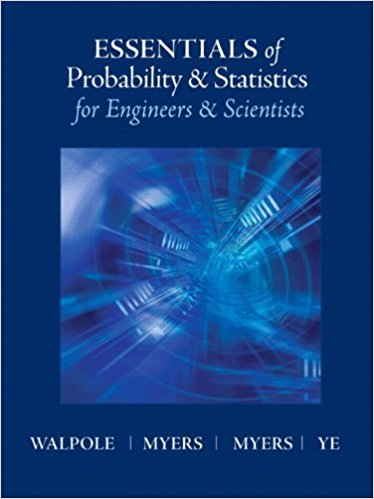×
×

# Solutions for Chapter 9: Factorial Experiments (Two or More Factors)## Full solutions for Essentials of Probability & Statistics for Engineers & Scientists | 1st Edition

ISBN: 9780321783738Solutions for Chapter 9: Factorial Experiments (Two or More Factors)

Solutions for Chapter 9
4 5 0 302 Reviews
25
4
##### ISBN: 9780321783738

This expansive textbook survival guide covers the following chapters and their solutions. Chapter 9: Factorial Experiments (Two or More Factors) includes 35 full step-by-step solutions. Essentials of Probability & Statistics for Engineers & Scientists was written by and is associated to the ISBN: 9780321783738. Since 35 problems in chapter 9: Factorial Experiments (Two or More Factors) have been answered, more than 15118 students have viewed full step-by-step solutions from this chapter. This textbook survival guide was created for the textbook: Essentials of Probability & Statistics for Engineers & Scientists, edition: 1.

Key Statistics Terms and definitions covered in this textbook
• Alternative hypothesis

In statistical hypothesis testing, this is a hypothesis other than the one that is being tested. The alternative hypothesis contains feasible conditions, whereas the null hypothesis speciies conditions that are under test

• Asymptotic relative eficiency (ARE)

Used to compare hypothesis tests. The ARE of one test relative to another is the limiting ratio of the sample sizes necessary to obtain identical error probabilities for the two procedures.

• Attribute control chart

Any control chart for a discrete random variable. See Variables control chart.

• Average run length, or ARL

The average number of samples taken in a process monitoring or inspection scheme until the scheme signals that the process is operating at a level different from the level in which it began.

• Axioms of probability

A set of rules that probabilities deined on a sample space must follow. See Probability

• Bimodal distribution.

A distribution with two modes

• C chart

An attribute control chart that plots the total number of defects per unit in a subgroup. Similar to a defects-per-unit or U chart.

• Categorical data

Data consisting of counts or observations that can be classiied into categories. The categories may be descriptive.

• Central limit theorem

The simplest form of the central limit theorem states that the sum of n independently distributed random variables will tend to be normally distributed as n becomes large. It is a necessary and suficient condition that none of the variances of the individual random variables are large in comparison to their sum. There are more general forms of the central theorem that allow ininite variances and correlated random variables, and there is a multivariate version of the theorem.

• Combination.

A subset selected without replacement from a set used to determine the number of outcomes in events and sample spaces.

• Continuous random variable.

A random variable with an interval (either inite or ininite) of real numbers for its range.

• Control chart

A graphical display used to monitor a process. It usually consists of a horizontal center line corresponding to the in-control value of the parameter that is being monitored and lower and upper control limits. The control limits are determined by statistical criteria and are not arbitrary, nor are they related to speciication limits. If sample points fall within the control limits, the process is said to be in-control, or free from assignable causes. Points beyond the control limits indicate an out-of-control process; that is, assignable causes are likely present. This signals the need to ind and remove the assignable causes.

• Cumulative distribution function

For a random variable X, the function of X deined as PX x ( ) ? that is used to specify the probability distribution.

• Curvilinear regression

An expression sometimes used for nonlinear regression models or polynomial regression models.

• Density function

Another name for a probability density function

• Eficiency

A concept in parameter estimation that uses the variances of different estimators; essentially, an estimator is more eficient than another estimator if it has smaller variance. When estimators are biased, the concept requires modiication.

• Enumerative study

A study in which a sample from a population is used to make inference to the population. See Analytic study

• Fraction defective control chart

See P chart

• Fractional factorial experiment

A type of factorial experiment in which not all possible treatment combinations are run. This is usually done to reduce the size of an experiment with several factors.

• Frequency distribution

An arrangement of the frequencies of observations in a sample or population according to the values that the observations take on

×